Flow Analysis Dataflow analysis Controlflow analysis Abstract interpretation

• Slides: 54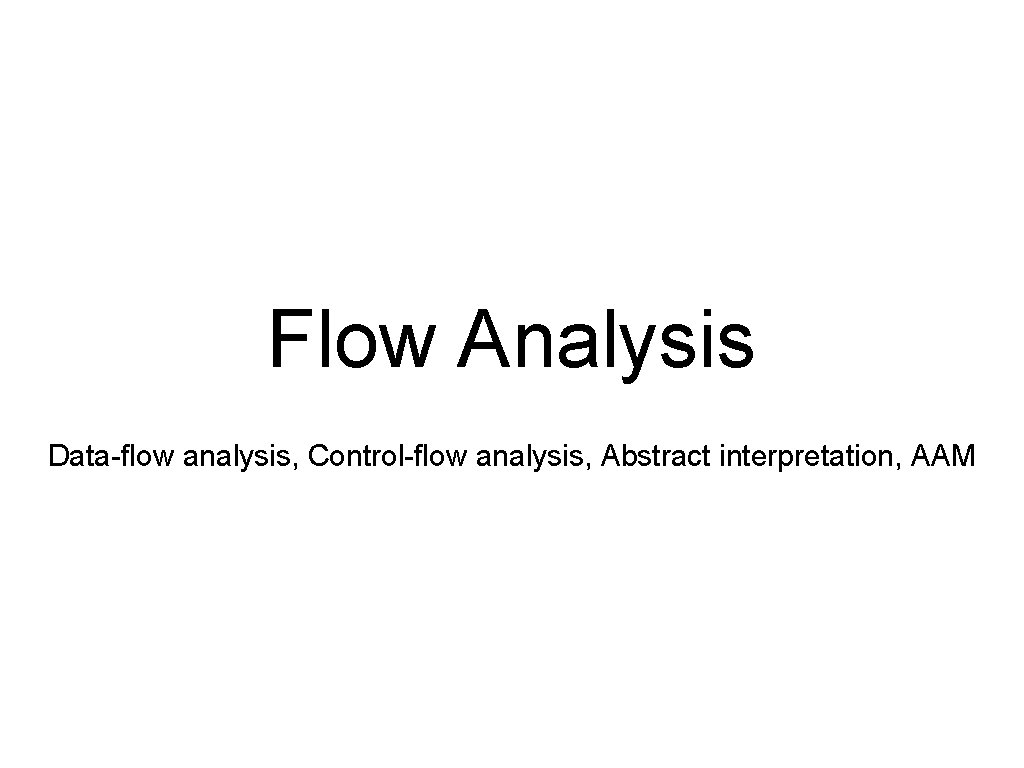Flow Analysis Data-flow analysis, Control-flow analysis, Abstract interpretation, AAM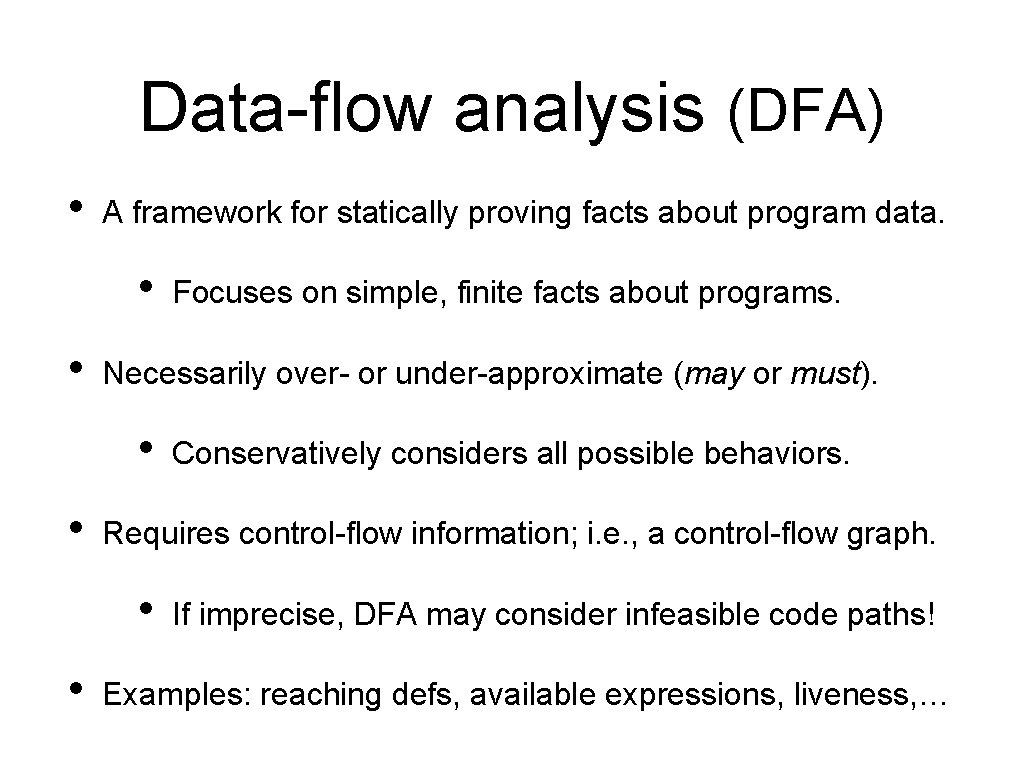Data-flow analysis (DFA) • A framework for statically proving facts about program data. • • Necessarily over- or under-approximate (may or must). • • Conservatively considers all possible behaviors. Requires control-flow information; i. e. , a control-flow graph. • • Focuses on simple, finite facts about programs. If imprecise, DFA may consider infeasible code paths! Examples: reaching defs, available expressions, liveness, …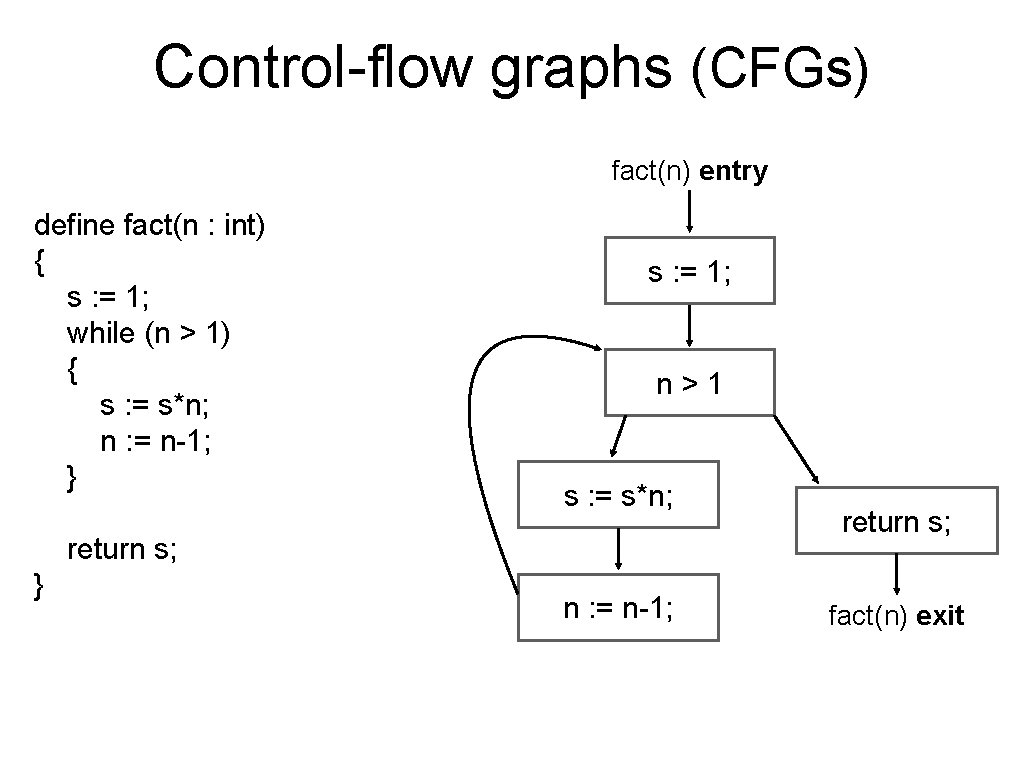Control-flow graphs (CFGs) fact(n) entry define fact(n : int) { s : = 1; while (n > 1) { s : = s*n; n : = n-1; } s : = 1; n>1 s : = s*n; return s; } n : = n-1; return s; fact(n) exit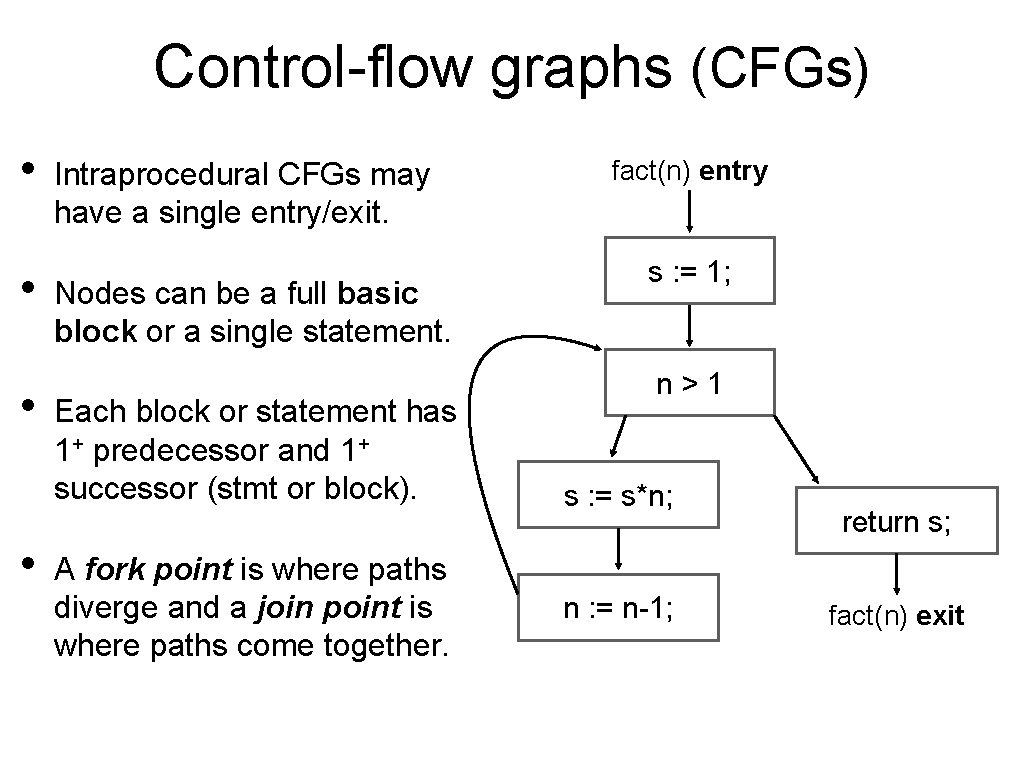Control-flow graphs (CFGs) • • Intraprocedural CFGs may have a single entry/exit. Nodes can be a full basic block or a single statement. Each block or statement has 1+ predecessor and 1+ successor (stmt or block). A fork point is where paths diverge and a join point is where paths come together. fact(n) entry s : = 1; n>1 s : = s*n; n : = n-1; return s; fact(n) exit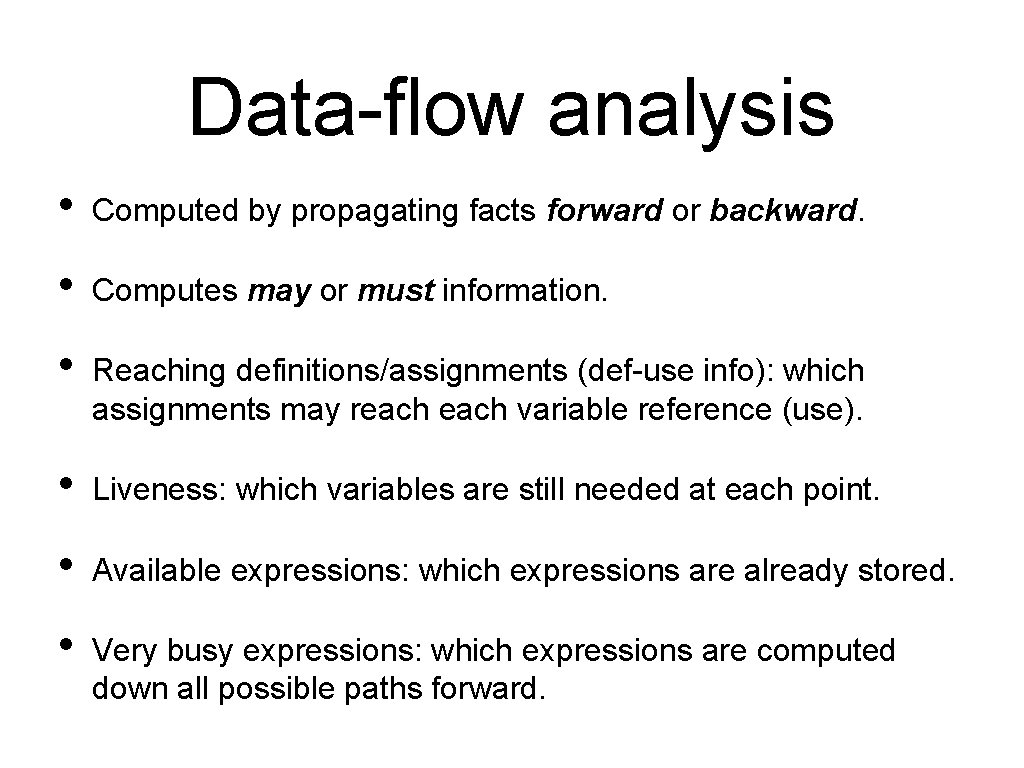Data-flow analysis • Computed by propagating facts forward or backward. • Computes may or must information. • Reaching definitions/assignments (def-use info): which assignments may reach variable reference (use). • Liveness: which variables are still needed at each point. • Available expressions: which expressions are already stored. • Very busy expressions: which expressions are computed down all possible paths forward.• Reaching defs, worklist algorithm Reaching definitions is a forward may analysis. • gen(s) yields facts generated by a statement s. • kill(s) yields facts invalidated by a statement s. • pred(s) and succ(s) yield sets of statements preceding/succ. • entry(s) = ∪ exit(s’); s’ ∈ pred(s) exit(s) = (entry(s) kill(s)) ∪ gen(s) • gen, kill, pred, succ, are fixed for each s; exit is monotonic in entry (if entry(s) grows, exit(s) grows); entry for exits of preds. • We iterate rules for entry/exit until reaching a fixed point.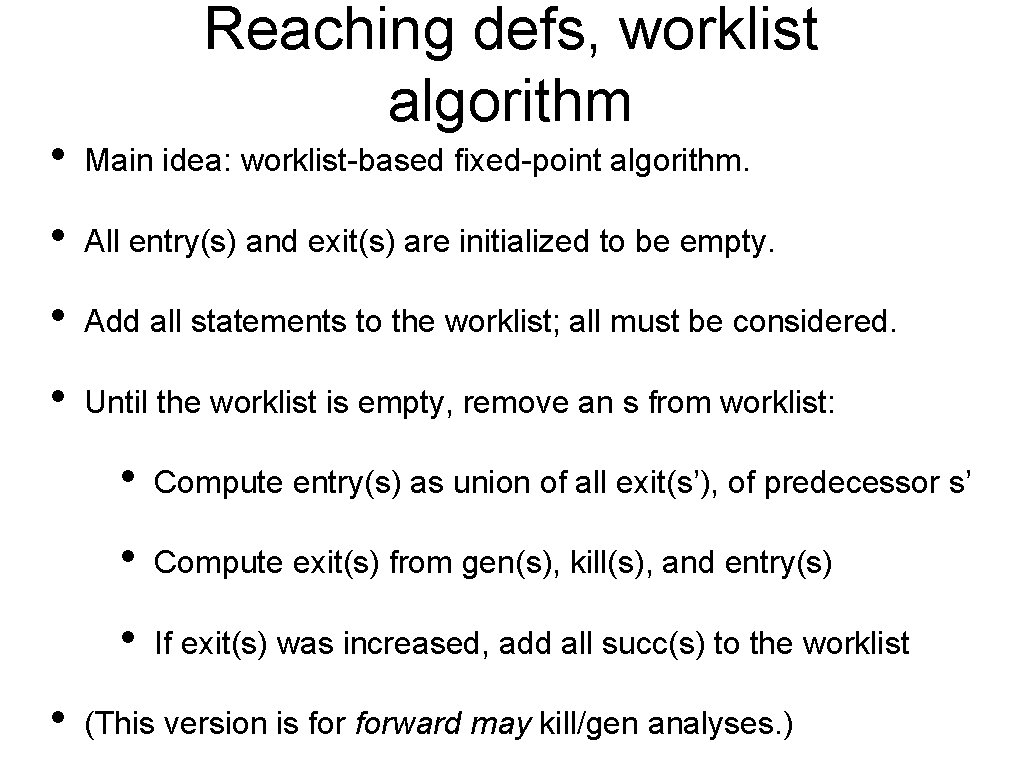Reaching defs, worklist algorithm • Main idea: worklist-based fixed-point algorithm. • All entry(s) and exit(s) are initialized to be empty. • Add all statements to the worklist; all must be considered. • Until the worklist is empty, remove an s from worklist: • • Compute entry(s) as union of all exit(s’), of predecessor s’ • Compute exit(s) from gen(s), kill(s), and entry(s) • If exit(s) was increased, add all succ(s) to the worklist (This version is forward may kill/gen analyses. )Reaching defs, worklist algorithm exit(s) = ∅ W = all statements s //worklist while W not empty: remove s from W entry(s) = ∪ s’∈pred(s)exit(s’) update = (entry(s) kill(s)) ∪ gen(s) if update != exit(s): exit(s) = update W = W ∪ succ(s) Forward may analysisReaching definitions analysis fact(n)0 entry Stmt s : = 1; 1 GEN (s, 1) KILL (s, *) n > 12 s : = s*n; 3 n : = n-1; 4 return s; 5 s : = 1; 1 (s, 3) (n, 4) (s, 1) (s, 3) (n, 0) (n, 4) s : = s*n; 3 n : = n-1; 4 return s; 5 fact(n) exit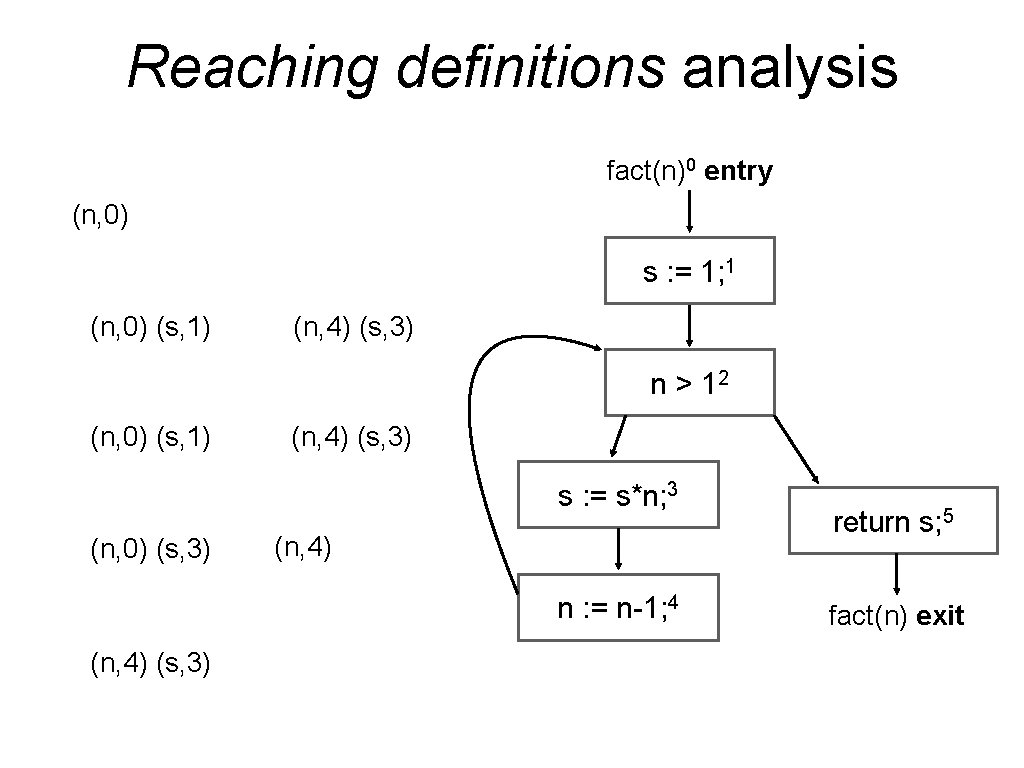Reaching definitions analysis fact(n)0 entry (n, 0) s : = 1; 1 (n, 0) (s, 1) (n, 4) (s, 3) n > 12 (n, 0) (s, 1) (n, 4) (s, 3) s : = s*n; 3 (n, 0) (s, 3) (n, 4) n : = n-1; 4 (n, 4) (s, 3) return s; 5 fact(n) exit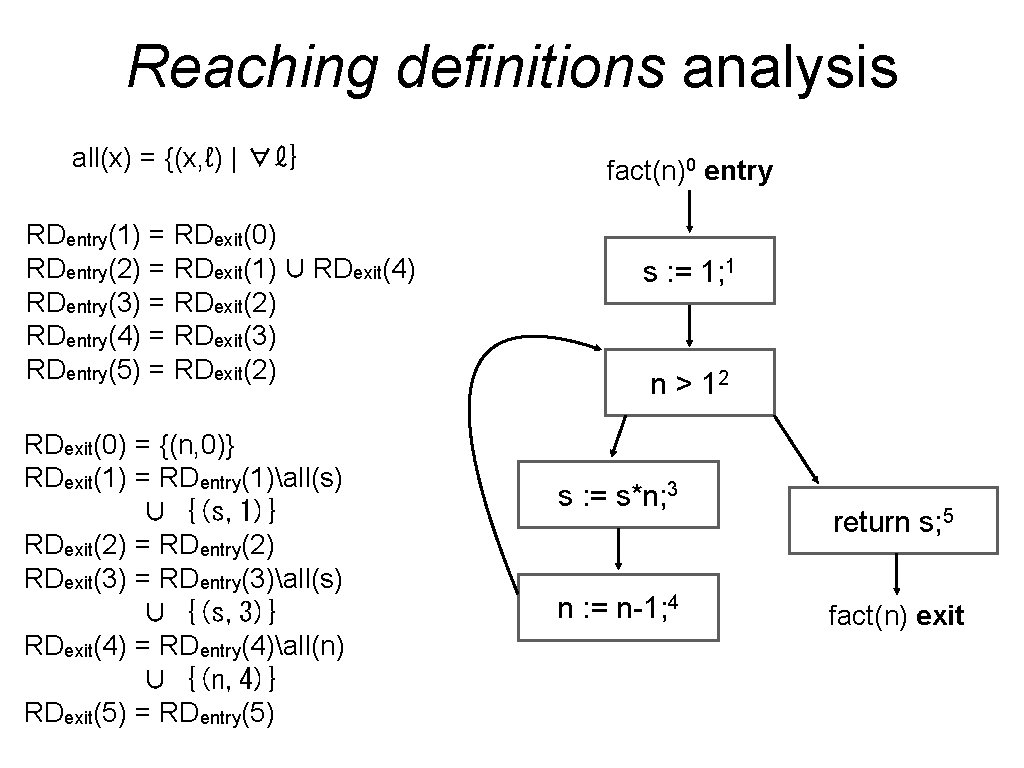Reaching definitions analysis all(x) = {(x, ℓ) | ∀ℓ} RDentry(1) = RDexit(0) RDentry(2) = RDexit(1) ∪ RDexit(4) RDentry(3) = RDexit(2) RDentry(4) = RDexit(3) RDentry(5) = RDexit(2) RDexit(0) = {(n, 0)} RDexit(1) = RDentry(1)all(s) ∪ {(s, 1)} RDexit(2) = RDentry(2) RDexit(3) = RDentry(3)all(s) ∪ {(s, 3)} RDexit(4) = RDentry(4)all(n) ∪ {(n, 4)} RDexit(5) = RDentry(5) fact(n)0 entry s : = 1; 1 n > 12 s : = s*n; 3 n : = n-1; 4 return s; 5 fact(n) exit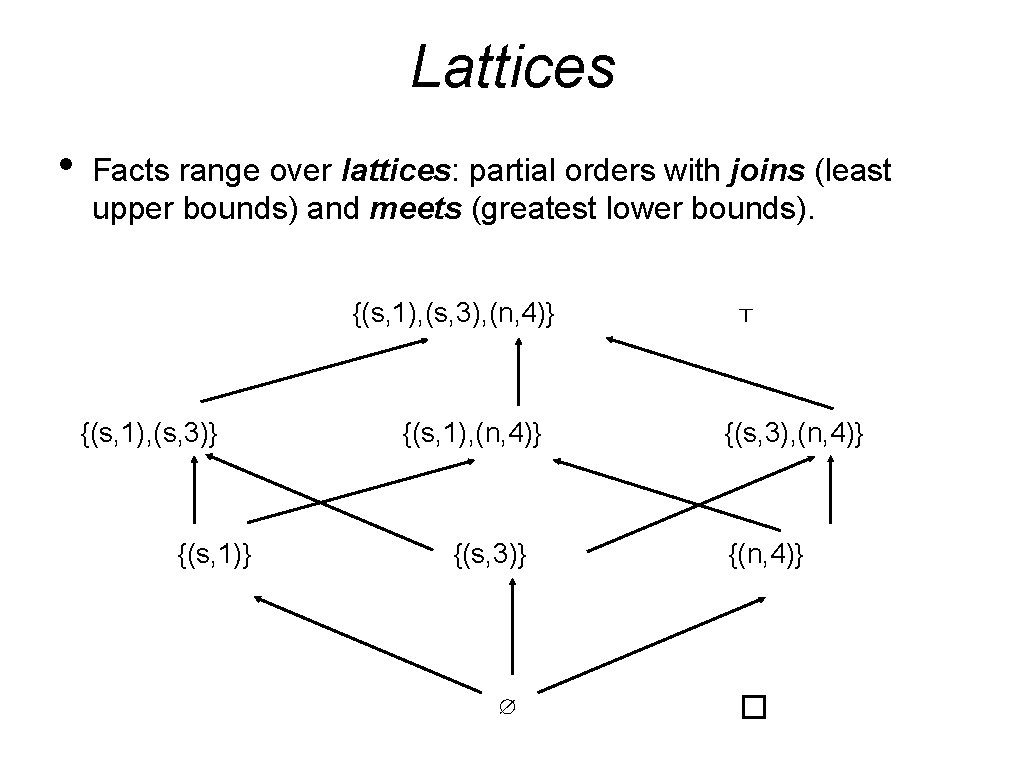Lattices • Facts range over lattices: partial orders with joins (least upper bounds) and meets (greatest lower bounds). {(s, 1), (s, 3), (n, 4)} {(s, 1), (s, 3)} {(s, 1), (n, 4)} {(s, 3)} ∅ ⊤ {(s, 3), (n, 4)} {(n, 4)} �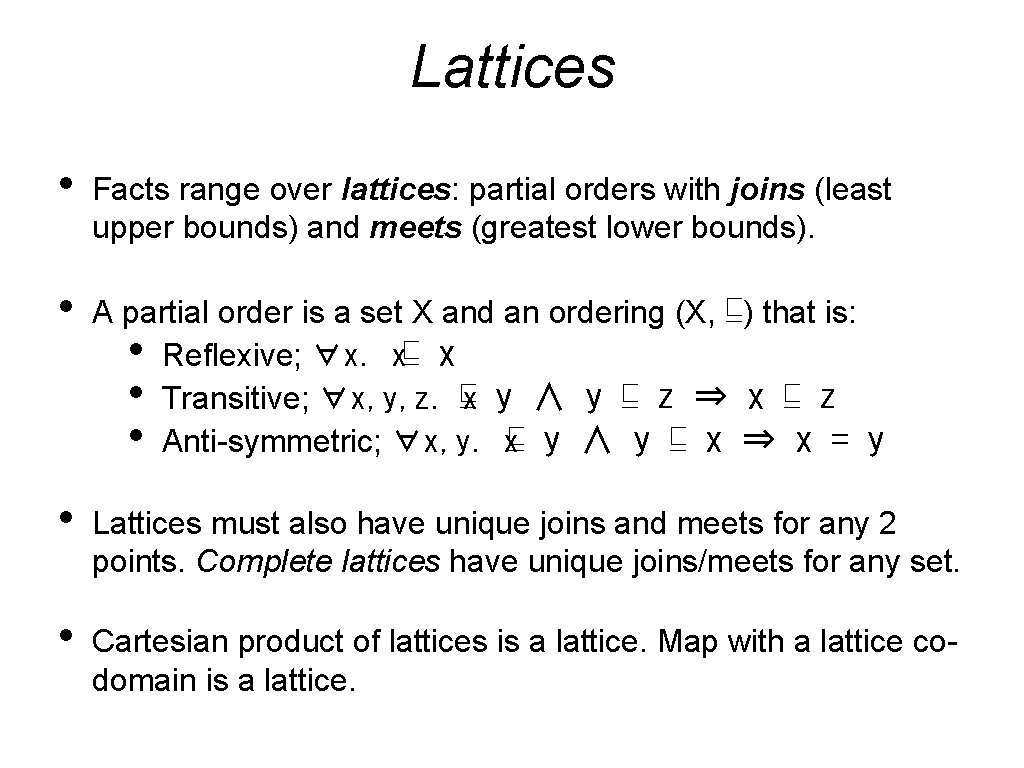Lattices • Facts range over lattices: partial orders with joins (least upper bounds) and meets (greatest lower bounds). • A partial order is a set X and an ordering (X, ⊑) that is: • Reflexive; ∀x. x⊑ x • Transitive; ∀x, y, z. ⊑x y ∧ y ⊑ z ⇒ x ⊑ z • Anti-symmetric; ∀x, y. x⊑ y ∧ y ⊑ x ⇒ x = y • Lattices must also have unique joins and meets for any 2 points. Complete lattices have unique joins/meets for any set. • Cartesian product of lattices is a lattice. Map with a lattice codomain is a lattice.Reaching definitions analysis • • The 11 sets RDexit(0), RDentry(1), RDexit(1), …RDexit(5), are defined in terms of one another. Written as a vector of sets: RD Our set of equations can be turned into a monotonic F: RD 0 ⊑ RD 1 ⇒ F(RD 0) ⊑ F(RD 1) • So that a satisfying vector of reachable defs is a fixed point: RD = F(RD) = Fn(�)for some n • For example, the join point would end up encoded as: F(…, RDexit(1), …, RDexit(4), …) = (…, RDexit(1) ∪ RDexit(4), …)Very busy expressions analysis • Computes a set of expressions that are computed down all paths forward before any subexpressions change value. • Assignments represent GEN for the right hand side and KILL for expressions containing the right hand side (assigned var). • Is a backward must data-flow analysis: • Propagates a set of computed expressions backward. • Computes the meet (GLB, intersection) of entry(s’) in s’∈succ(s) at each fork point to obtain exit(s).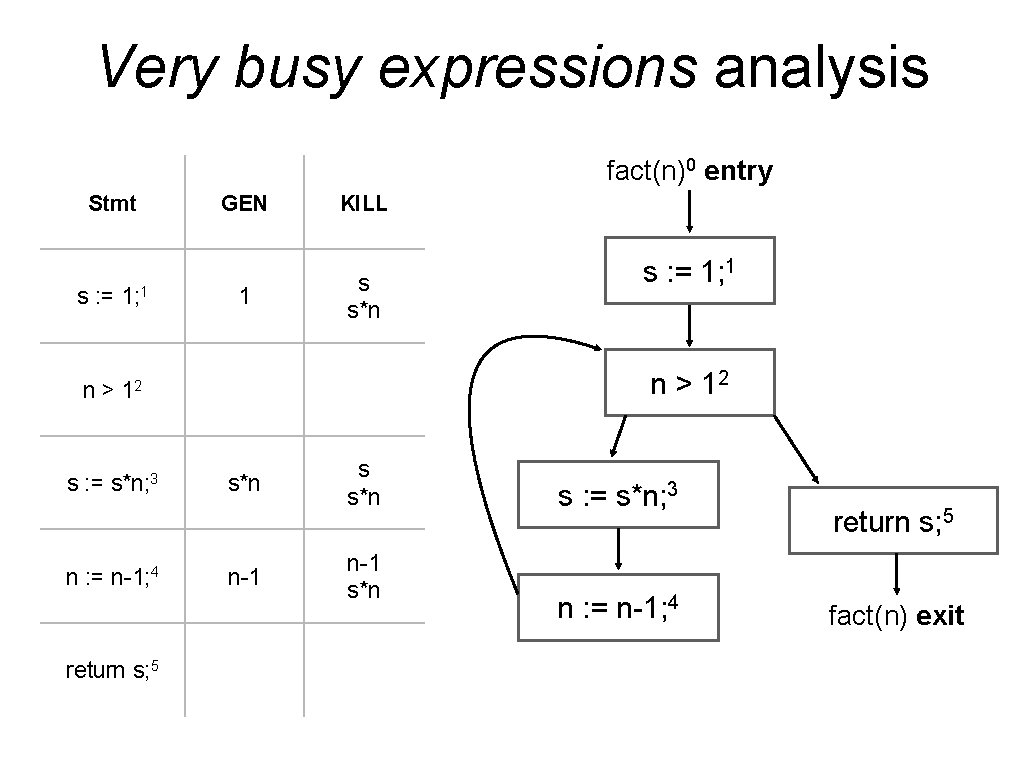Very busy expressions analysis fact(n)0 entry Stmt s : = 1; 1 GEN 1 KILL s s*n n > 12 s : = s*n; 3 n : = n-1; 4 return s; 5 s : = 1; 1 s*n n-1 s*n s : = s*n; 3 n : = n-1; 4 return s; 5 fact(n) exitVery busy expressions analysis fact(n)0 entry 1 s : = 1; 1 ∅ n > 12 ∅ s*n, n-1 ∅ s : = s*n; 3 n-1 ∅ n : = n-1; 4 ∅ return s; 5 fact(n) exit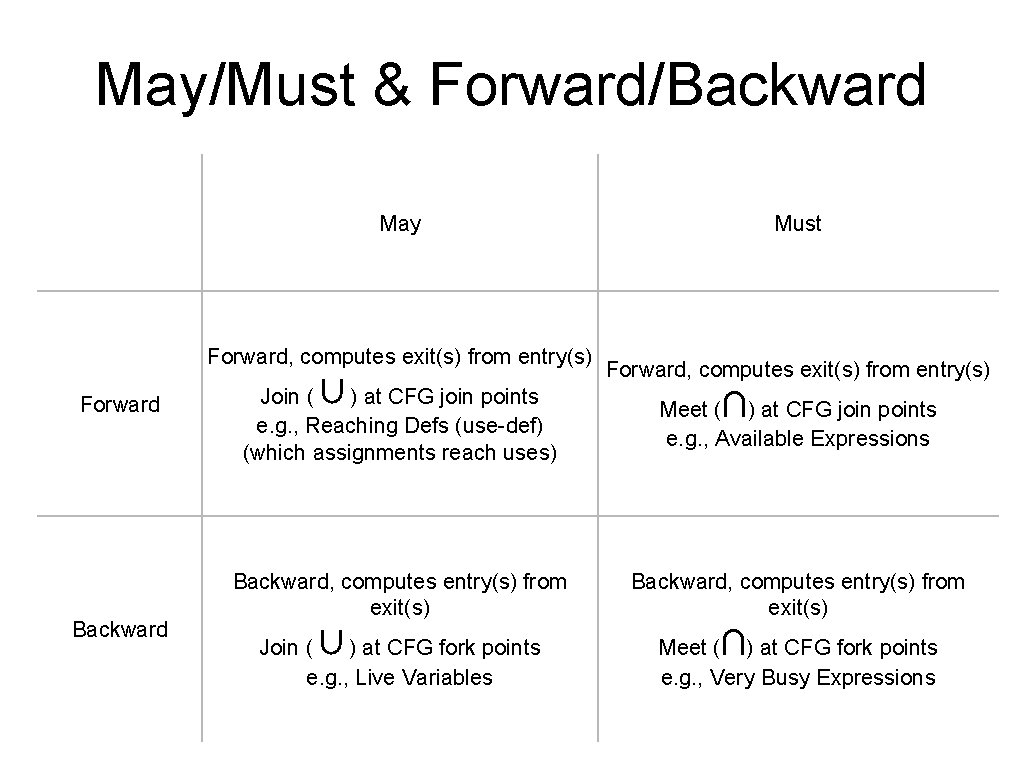May/Must & Forward/Backward May Forward, computes exit(s) from entry(s) Forward Backward ∪ Must Forward, computes exit(s) from entry(s) Join ( ) at CFG join points e. g. , Reaching Defs (use-def) (which assignments reach uses) Meet ( ) at CFG join points e. g. , Available Expressions Backward, computes entry(s) from exit(s) ∪ Join ( ) at CFG fork points e. g. , Live Variables ∩ ∩ Meet ( ) at CFG fork points e. g. , Very Busy Expressions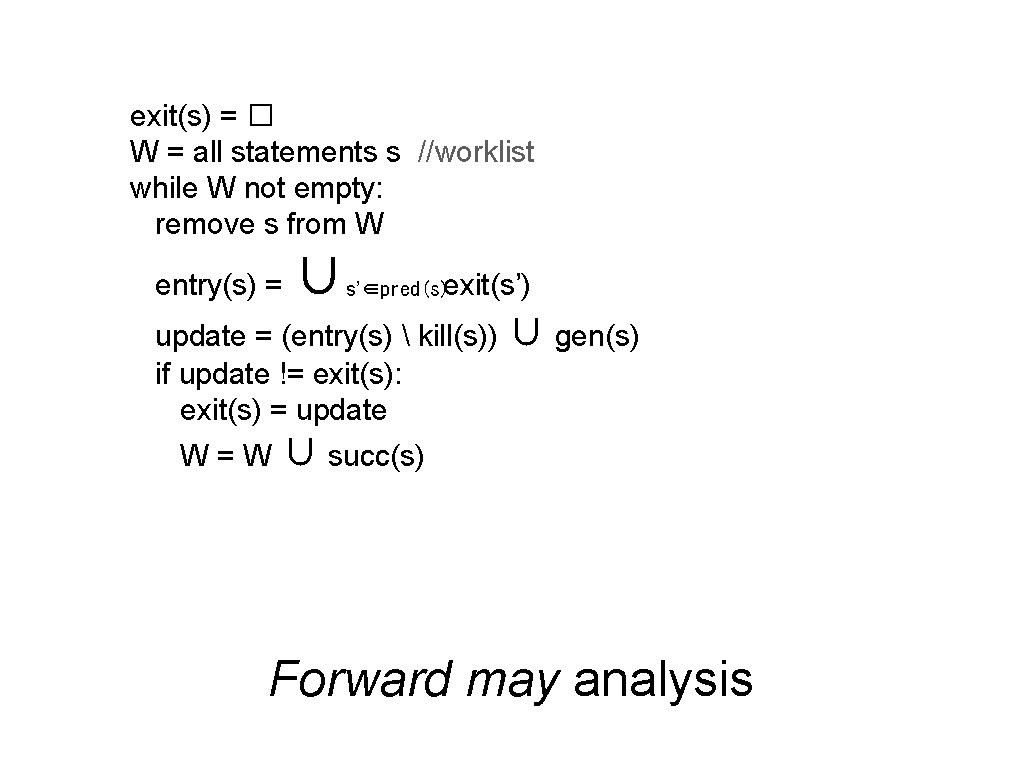exit(s) = � W = all statements s //worklist while W not empty: remove s from W entry(s) = ∪ s’∈pred(s)exit(s’) update = (entry(s) kill(s)) ∪ gen(s) if update != exit(s): exit(s) = update W = W ∪ succ(s) Forward may analysis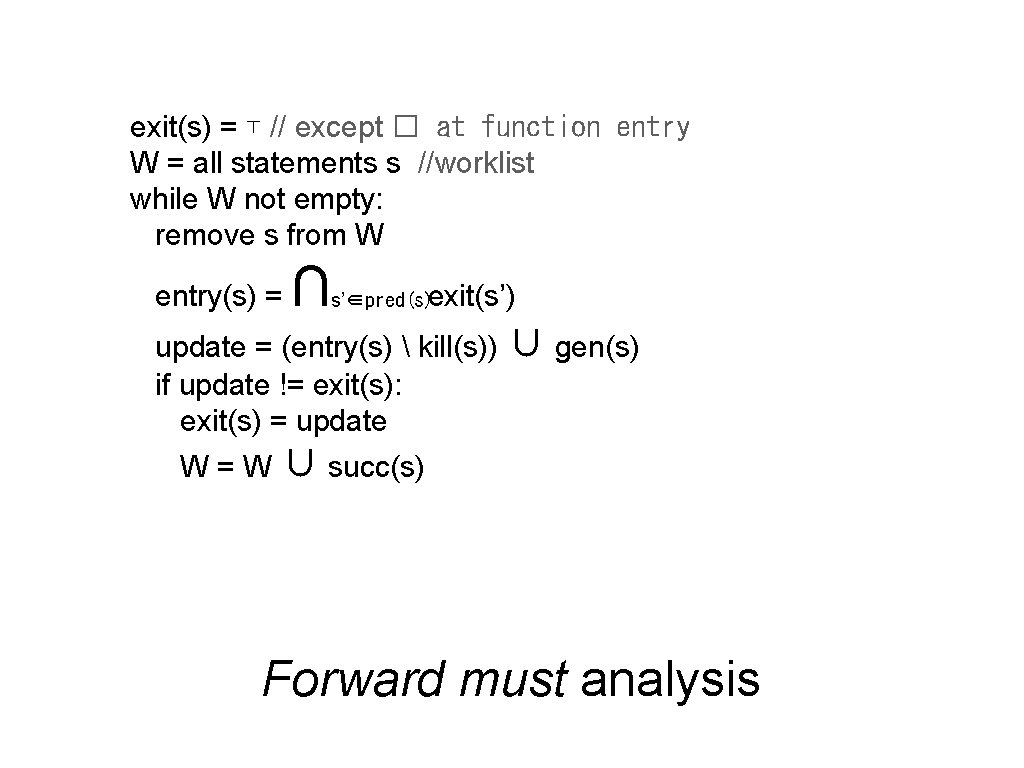exit(s) = ⊤ // except � at function entry W = all statements s //worklist while W not empty: remove s from W entry(s) = ∩ s’∈pred(s)exit(s’) update = (entry(s) kill(s)) ∪ gen(s) if update != exit(s): exit(s) = update W = W ∪ succ(s) Forward must analysis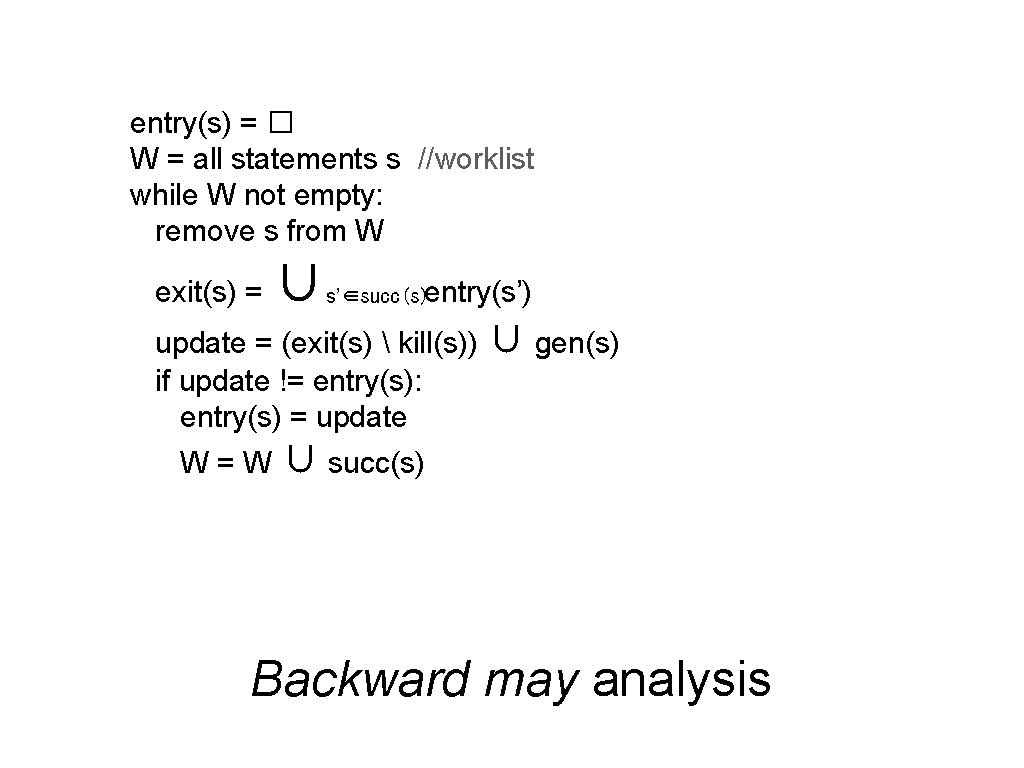entry(s) = � W = all statements s //worklist while W not empty: remove s from W exit(s) = ∪ s’∈succ(s)entry(s’) update = (exit(s) kill(s)) ∪ gen(s) if update != entry(s): entry(s) = update W = W ∪ succ(s) Backward may analysisentry(s) = ⊤ // except � at function exit W = all statements s //worklist while W not empty: remove s from W exit(s) = ∩ s’∈succ(s)entry(s’) update = (exit(s) kill(s)) ∪ gen(s) if update != entry(s): entry(s) = update W = W ∪ succ(s) Backward must analysis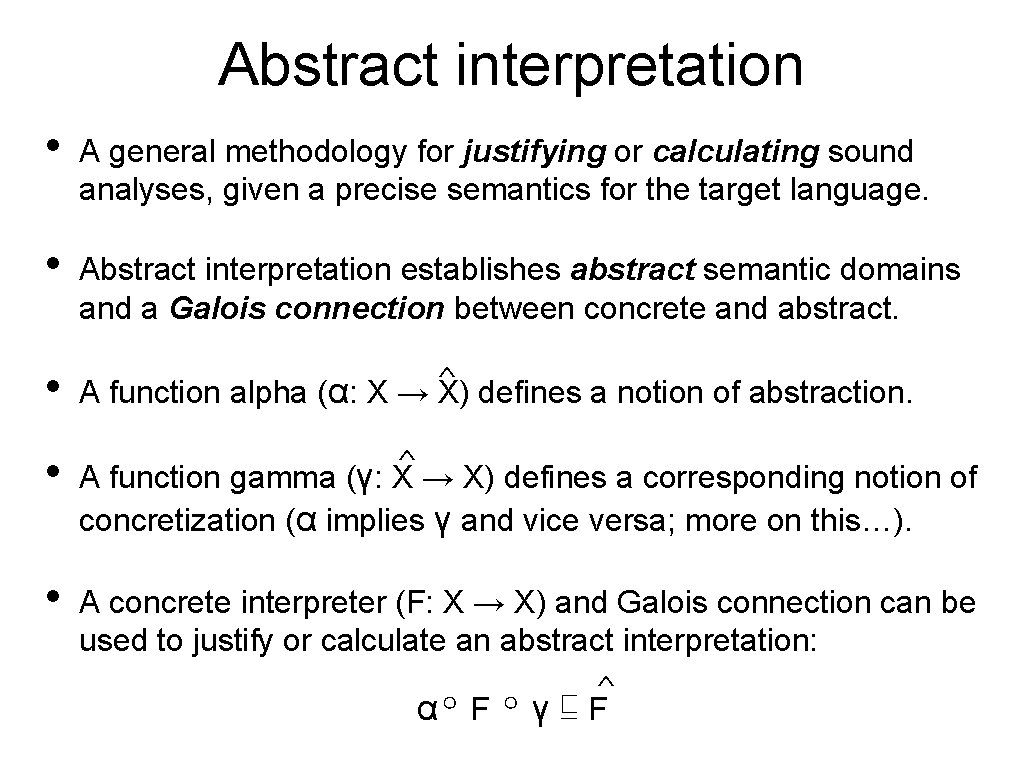Abstract interpretation • A general methodology for justifying or calculating sound analyses, given a precise semantics for the target language. • Abstract interpretation establishes abstract semantic domains and a Galois connection between concrete and abstract. • ^ A function alpha (α: X → X) defines a notion of abstraction. ^ • A function gamma (γ: X → X) defines a corresponding notion of concretization (α implies γ and vice versa; more on this…). • A concrete interpreter (F: X → X) and Galois connection can be used to justify or calculate an abstract interpretation: ^ α∘ F ∘ γ ⊑ FAbstraction/Concretization (Galois) ^ α( x ) ⊑ x if and only if x⊑ γ ( x^)Abstraction/Concretization (Galois conn. ) γ γ( x^ ) ^ ⊑ ⊑ x x α( x ) α X ^ X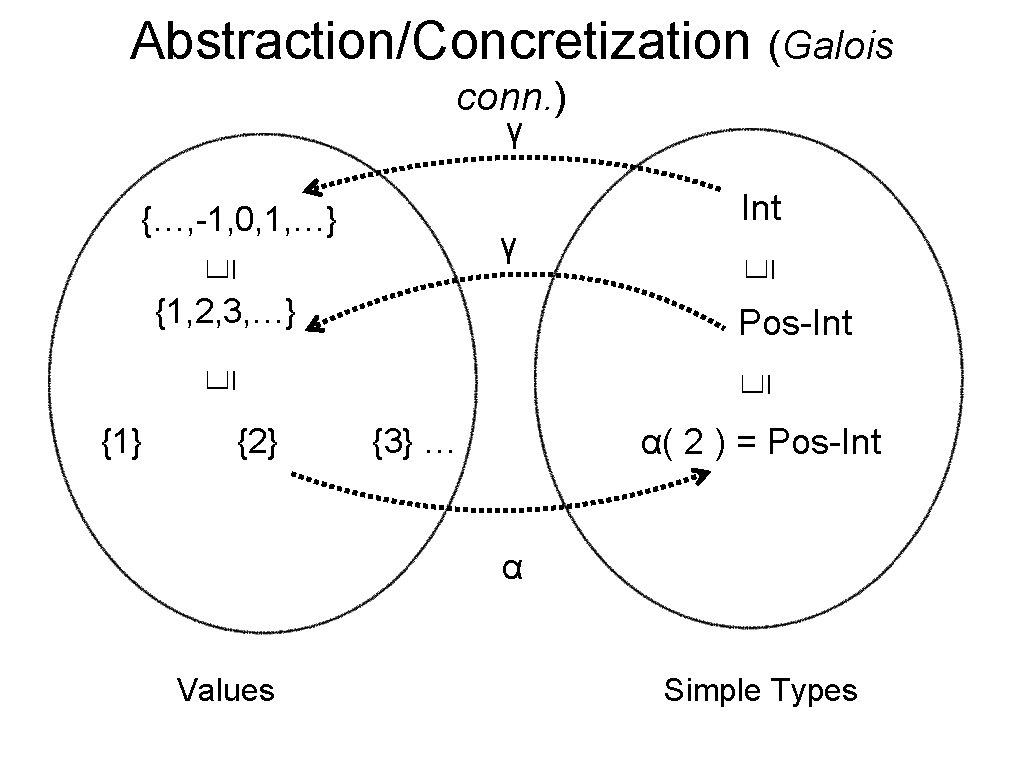Abstraction/Concretization (Galois conn. ) γ ⊑ γ {1, 2, 3, …} Pos-Int {2} ⊑ ⊑ {1} Int ⊑ {…, -1, 0, 1, …} α( 2 ) = Pos-Int {3} … α Values Simple Types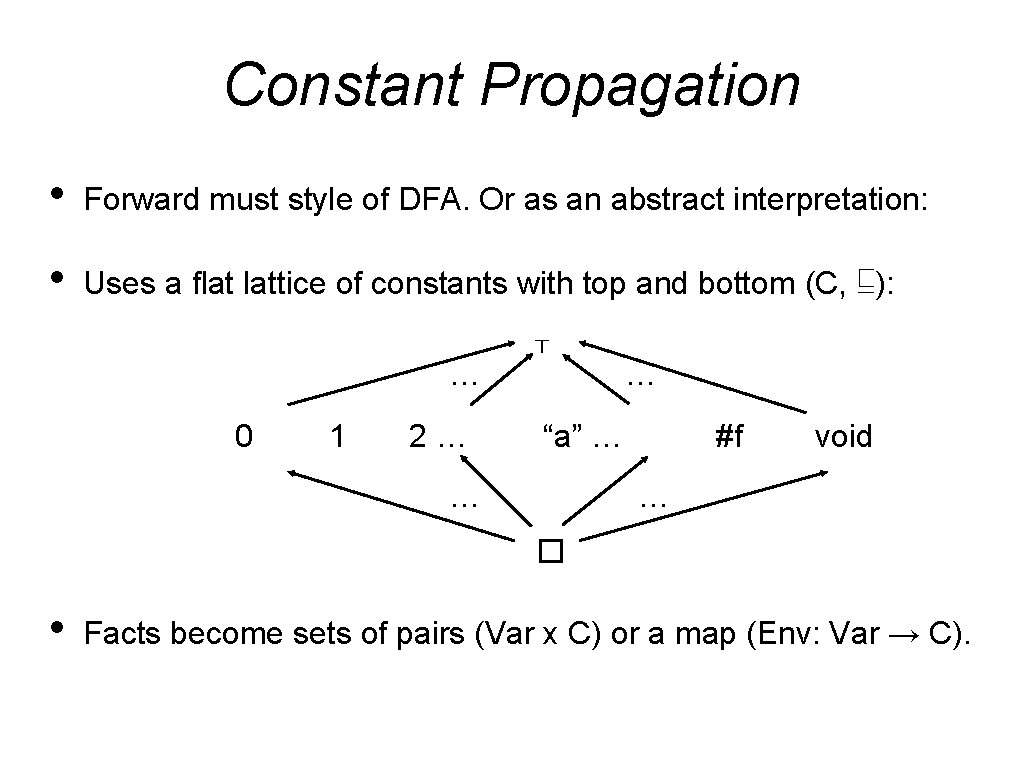Constant Propagation • Forward must style of DFA. Or as an abstract interpretation: • Uses a flat lattice of constants with top and bottom (C, ⊑): … 0 1 2… ⊤ … “a” … … #f void … � • Facts become sets of pairs (Var x C) or a map (Env: Var → C).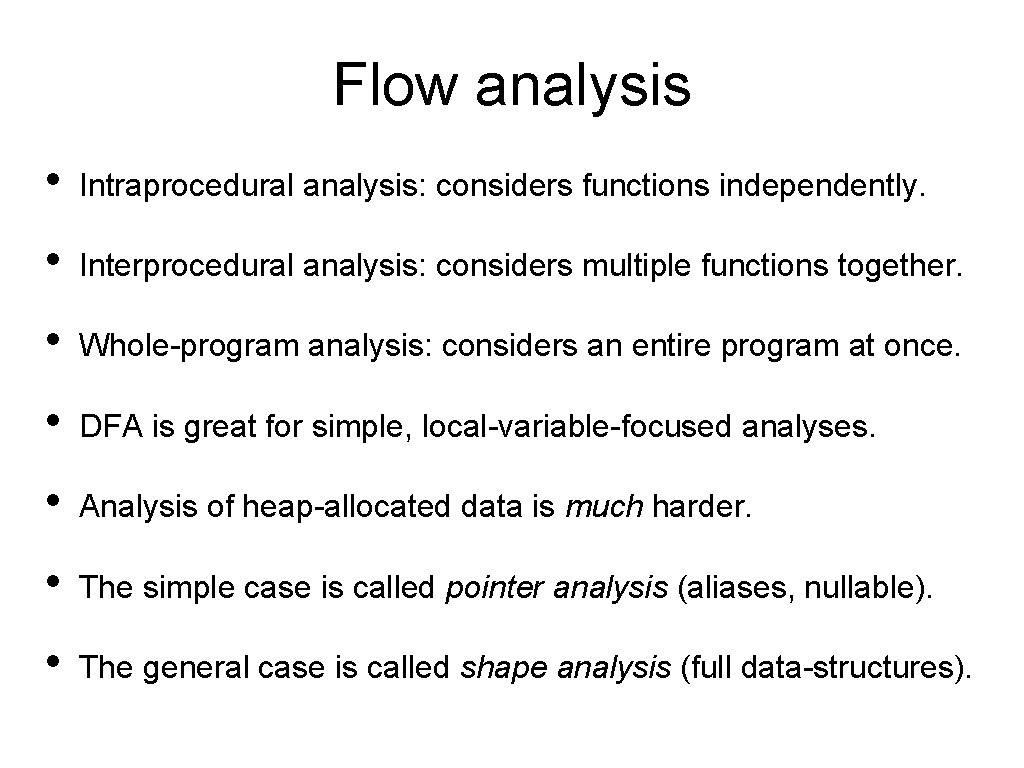Flow analysis • Intraprocedural analysis: considers functions independently. • Interprocedural analysis: considers multiple functions together. • Whole-program analysis: considers an entire program at once. • DFA is great for simple, local-variable-focused analyses. • Analysis of heap-allocated data is much harder. • The simple case is called pointer analysis (aliases, nullable). • The general case is called shape analysis (full data-structures).What about Scheme or ANF/CPS IRs?Depends on call sites for the lambda that binds it. (lambda (k f x y) (let ([a (prim + x y)]) (f k a))) What value can f be? Data-flow depends upon control-flow.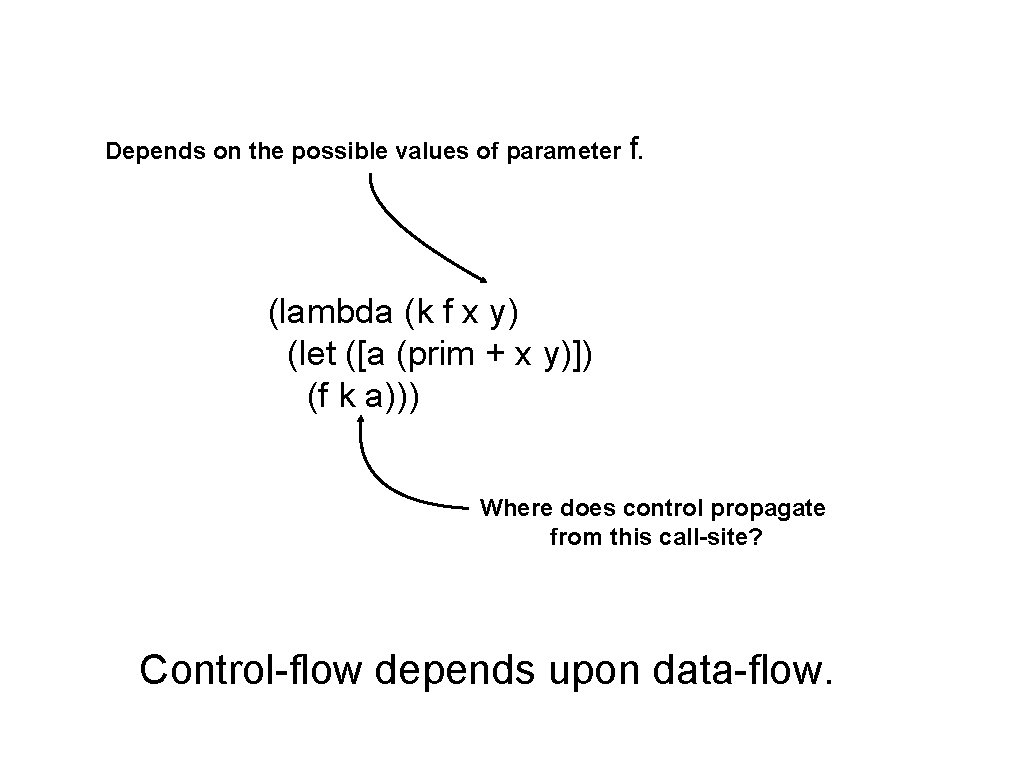Depends on the possible values of parameter f. (lambda (k f x y) (let ([a (prim + x y)]) (f k a))) Where does control propagate from this call-site? Control-flow depends upon data-flow.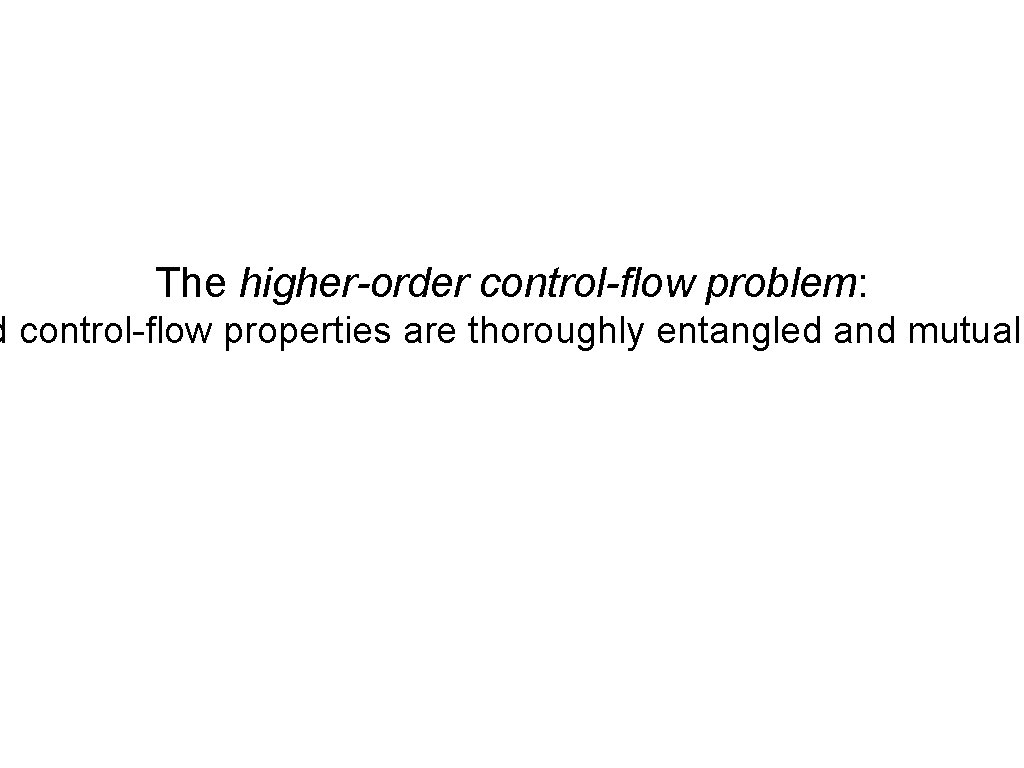The higher-order control-flow problem: d control-flow properties are thoroughly entangled and mutuall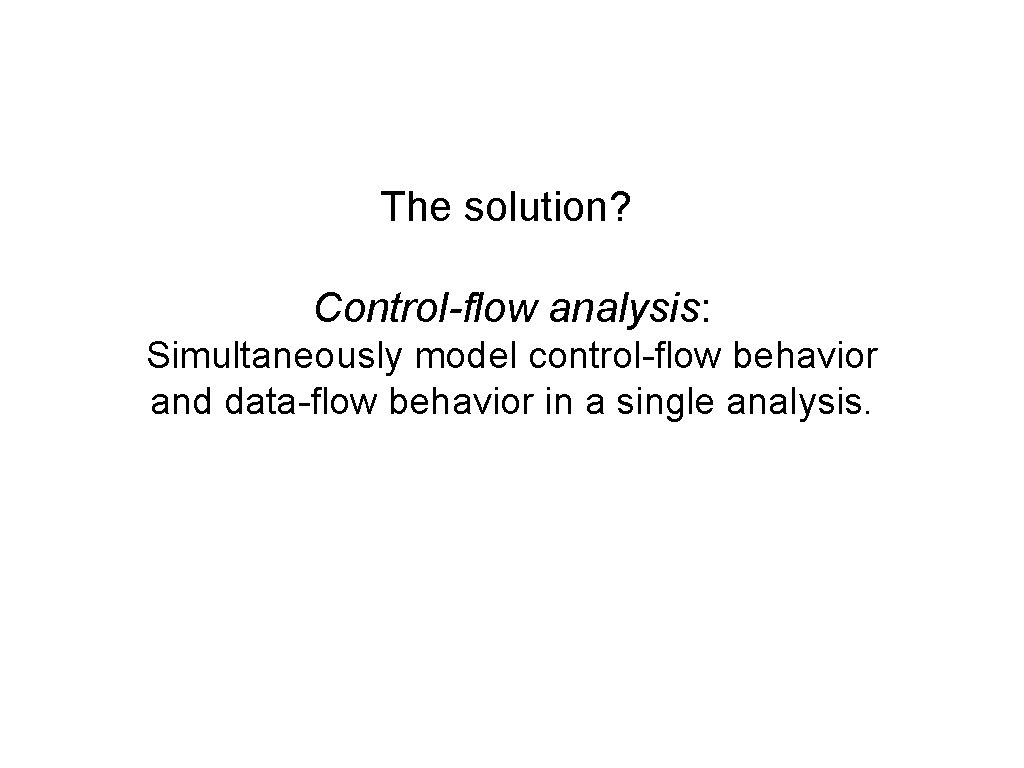The solution? Control-flow analysis: Simultaneously model control-flow behavior and data-flow behavior in a single analysis.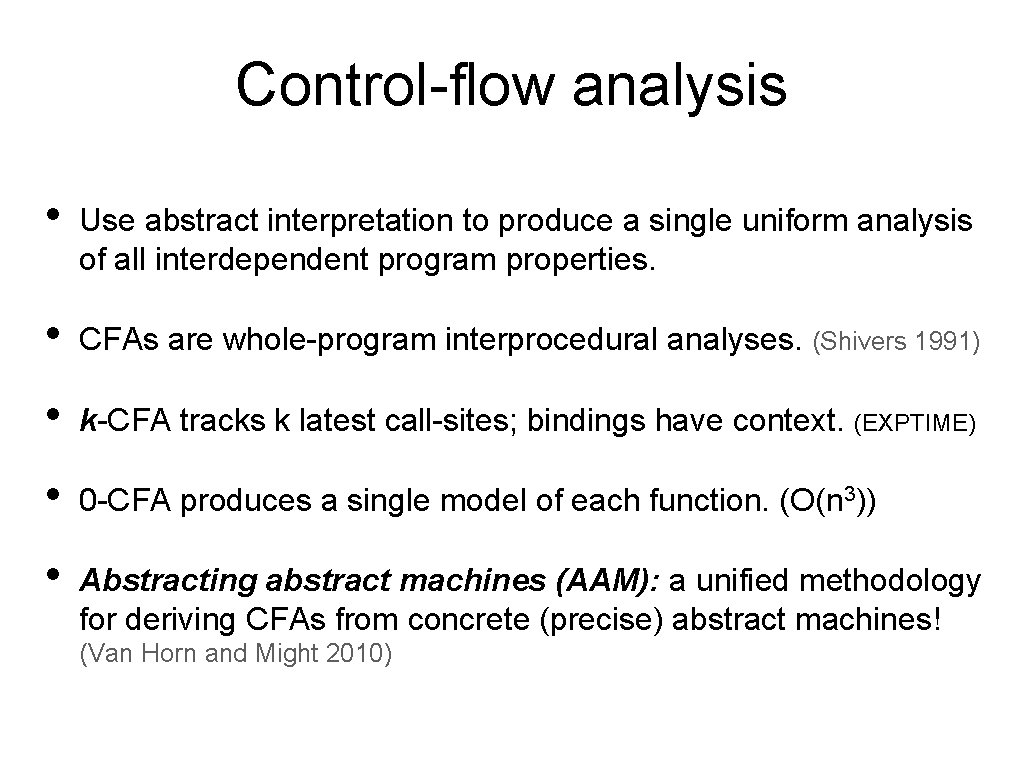Control-flow analysis • Use abstract interpretation to produce a single uniform analysis of all interdependent program properties. • CFAs are whole-program interprocedural analyses. (Shivers 1991) • k-CFA tracks k latest call-sites; bindings have context. (EXPTIME) • 0 -CFA produces a single model of each function. (O(n 3)) • Abstracting abstract machines (AAM): a unified methodology for deriving CFAs from concrete (precise) abstract machines! (Van Horn and Might 2010)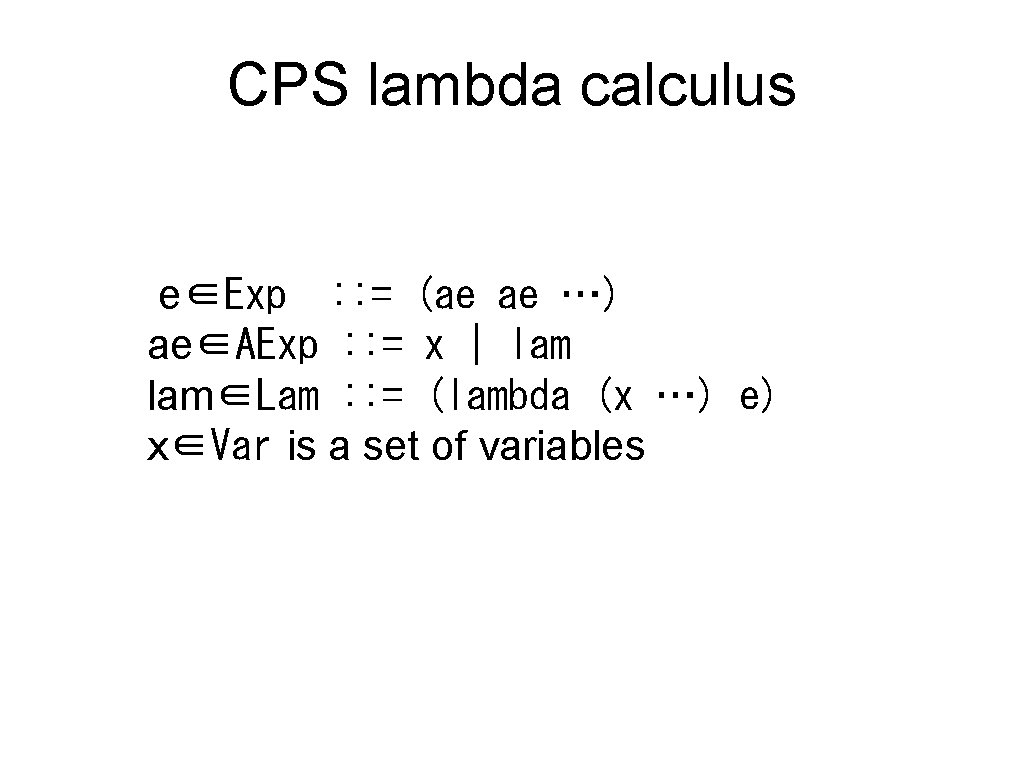CPS lambda calculus e∈Exp : : = (ae ae …) ae∈AExp : : = x | lam∈Lam : : = (lambda (x …) e) x∈Var is a set of variables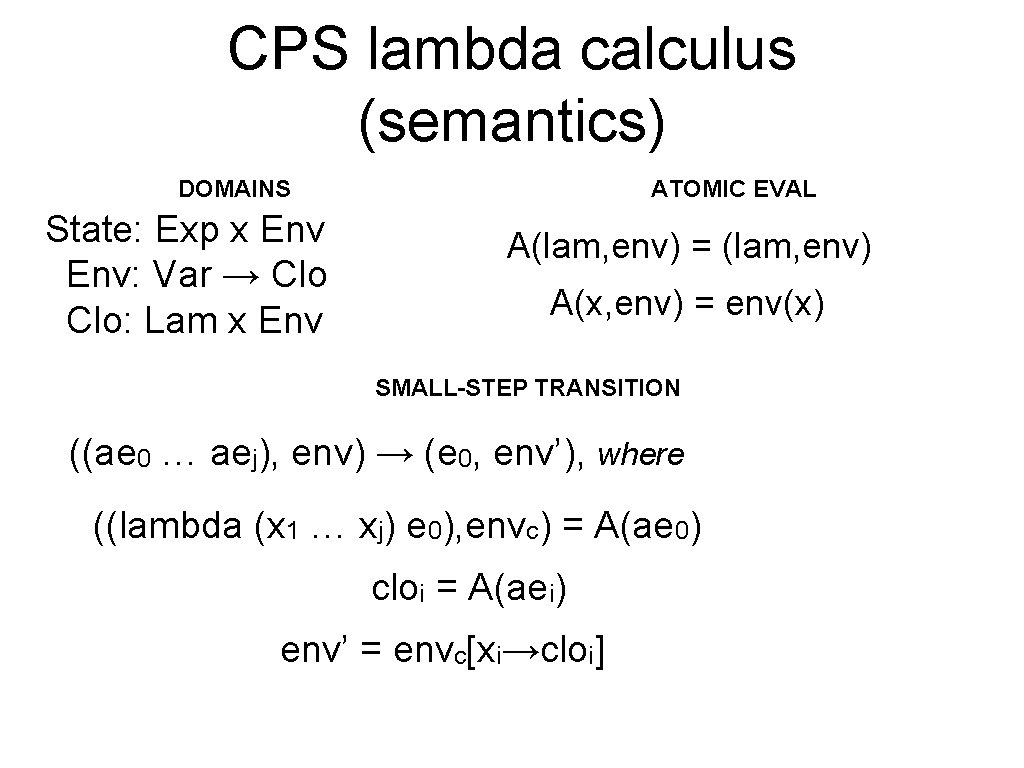CPS lambda calculus (semantics) DOMAINS State: Exp x Env: Var → Clo: Lam x Env ATOMIC EVAL A(lam, env) = (lam, env) A(x, env) = env(x) SMALL-STEP TRANSITION ((ae 0 … aej), env) → (e 0, env’), where ((lambda (x 1 … xj) e 0), envc) = A(ae 0) cloi = A(aei) env’ = envc[xi→cloi]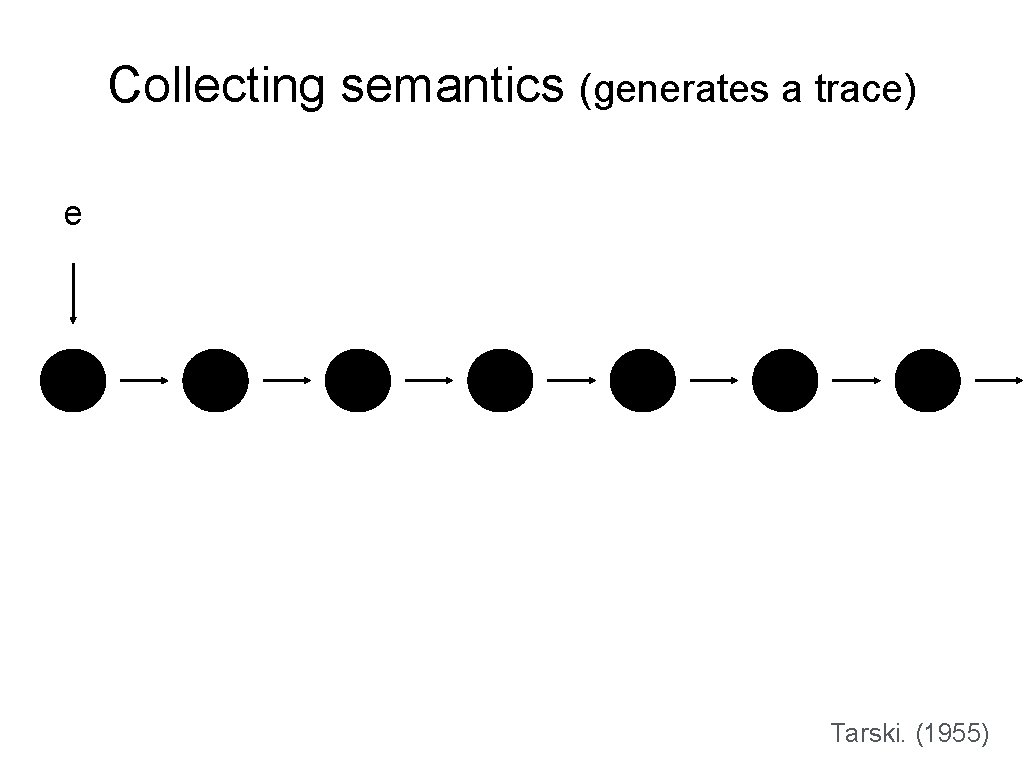Collecting semantics (generates a trace) e Tarski. (1955)Exp x Env: Var → Clo Might. Abstract interpreters for free. (2010)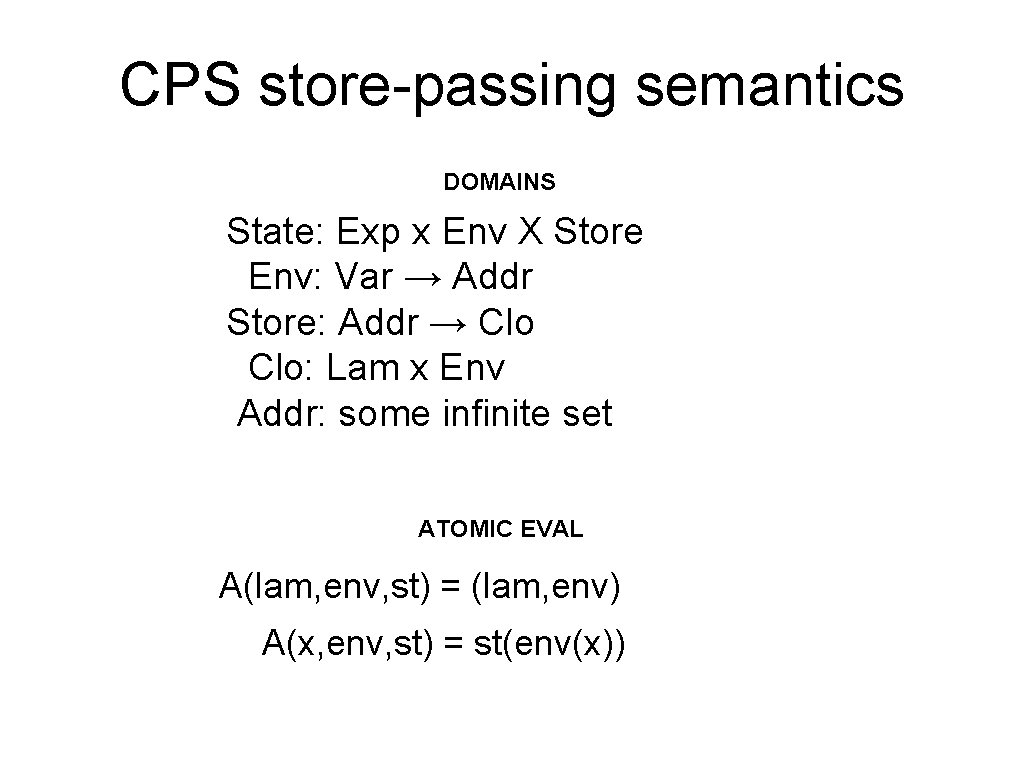CPS store-passing semantics DOMAINS State: Exp x Env X Store Env: Var → Addr Store: Addr → Clo: Lam x Env Addr: some infinite set ATOMIC EVAL A(lam, env, st) = (lam, env) A(x, env, st) = st(env(x))CPS store-passing semantics SMALL-STEP TRANSITION ((ae 0 … aej), env, st) → (e 0, env’, st’), where ((lambda (x 1 … xj) e 0), envc) = A(ae 0) cloi = A(aei) env’ = envc[xi→alloc(xi)] st’ = st[alloc(xi)→cloi] alloc(xi) = fresh address = xi (yields 0 -CFA)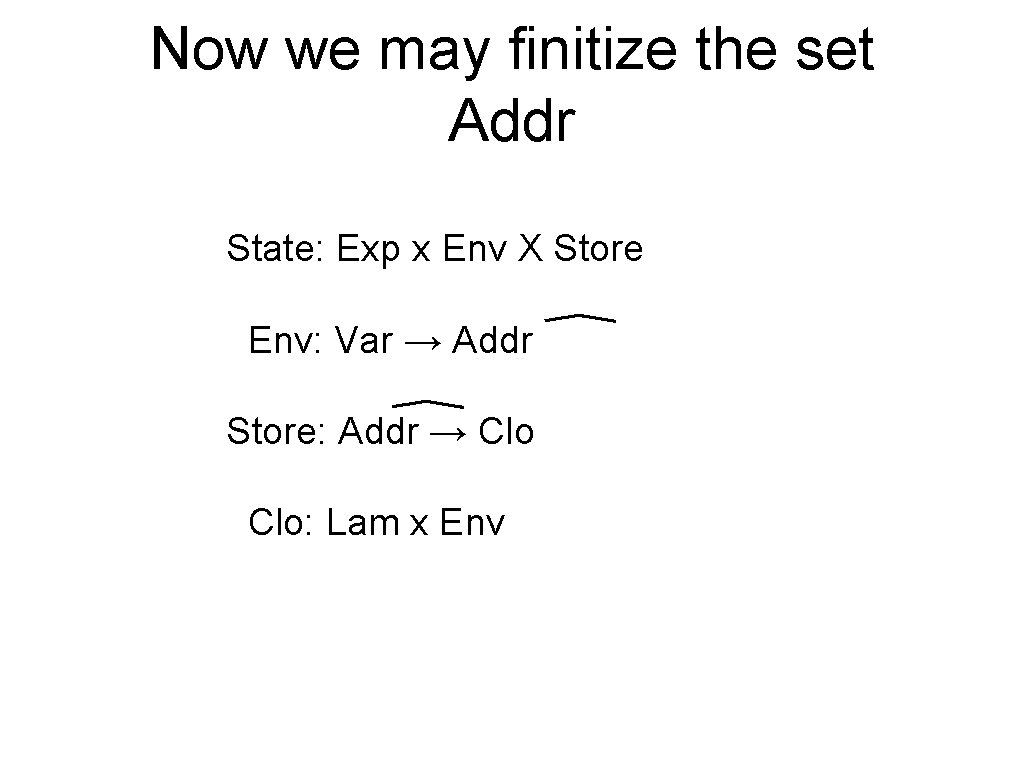Now we may finitize the set Addr State: Exp x Env X Store Env: Var → Addr Store: Addr → Clo: Lam x Env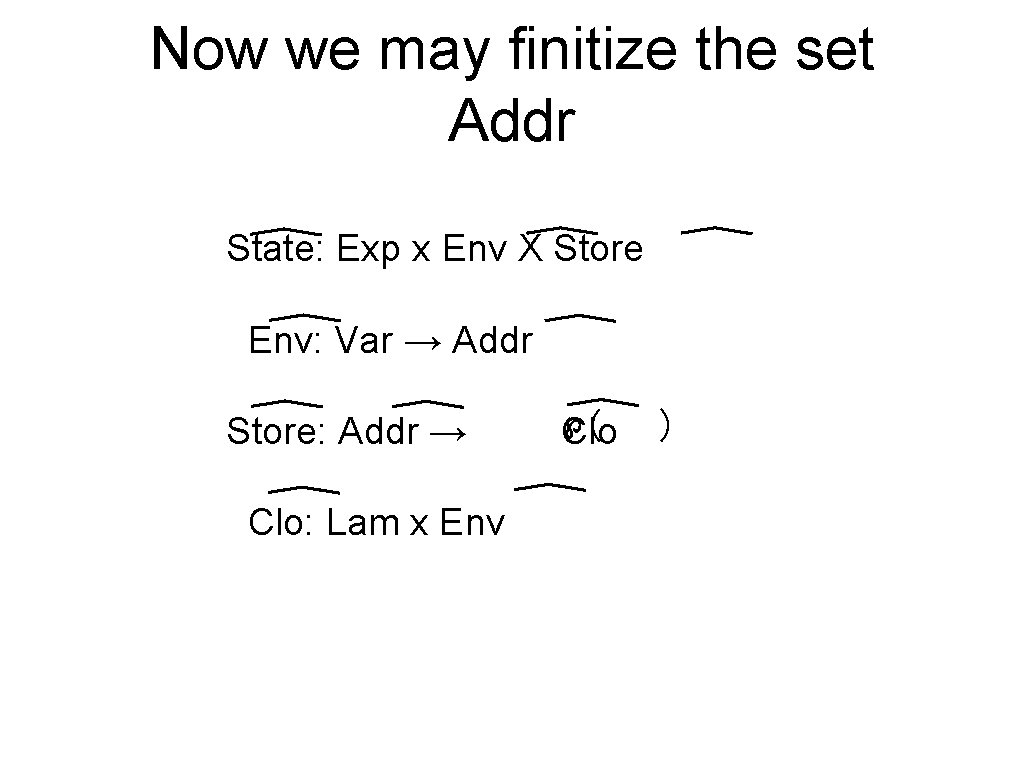Now we may finitize the set Addr State: Exp x Env X Store Env: Var → Addr Store: Addr → Clo: Lam x Env ℘( Clo )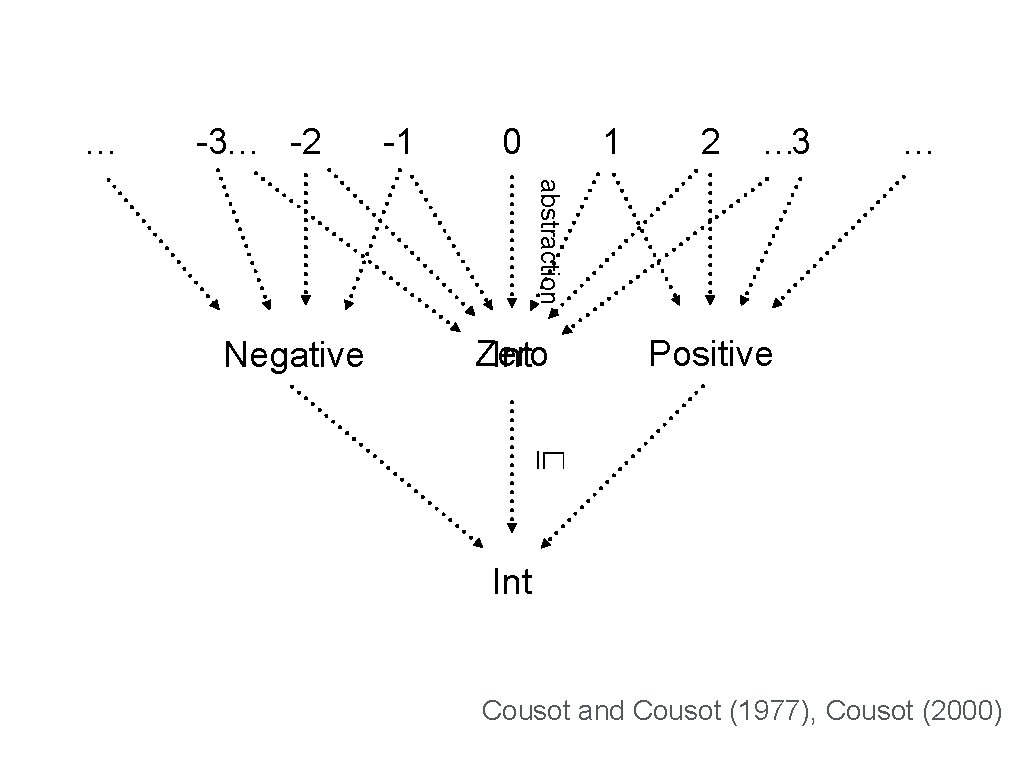… -3… -2 -1 0 1 2 … 3 … abstraction Negative Zero Int Positive ⊑ Int Cousot and Cousot (1977), Cousot (2000)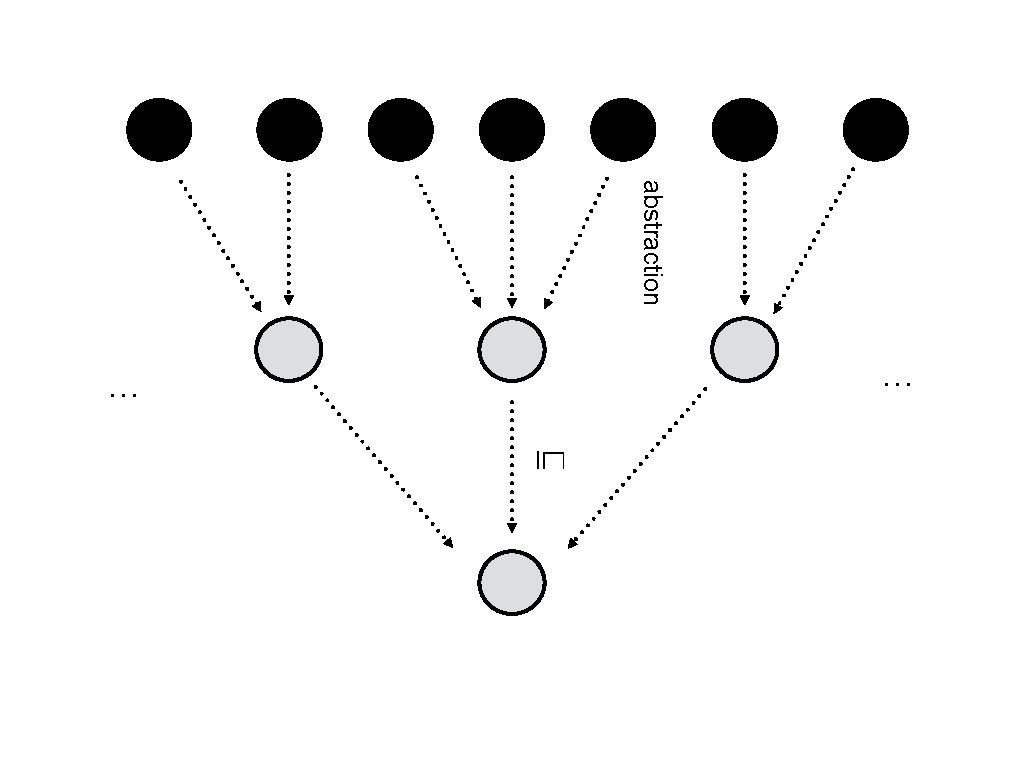abstraction … … ⊑Abstract abstract machines ( (f x) , [x → ax, y → ay, f → a f] , [ax → {Int}, ay → {Int}, af → {(λ (w) e 1), (λ (z) e 2)}] )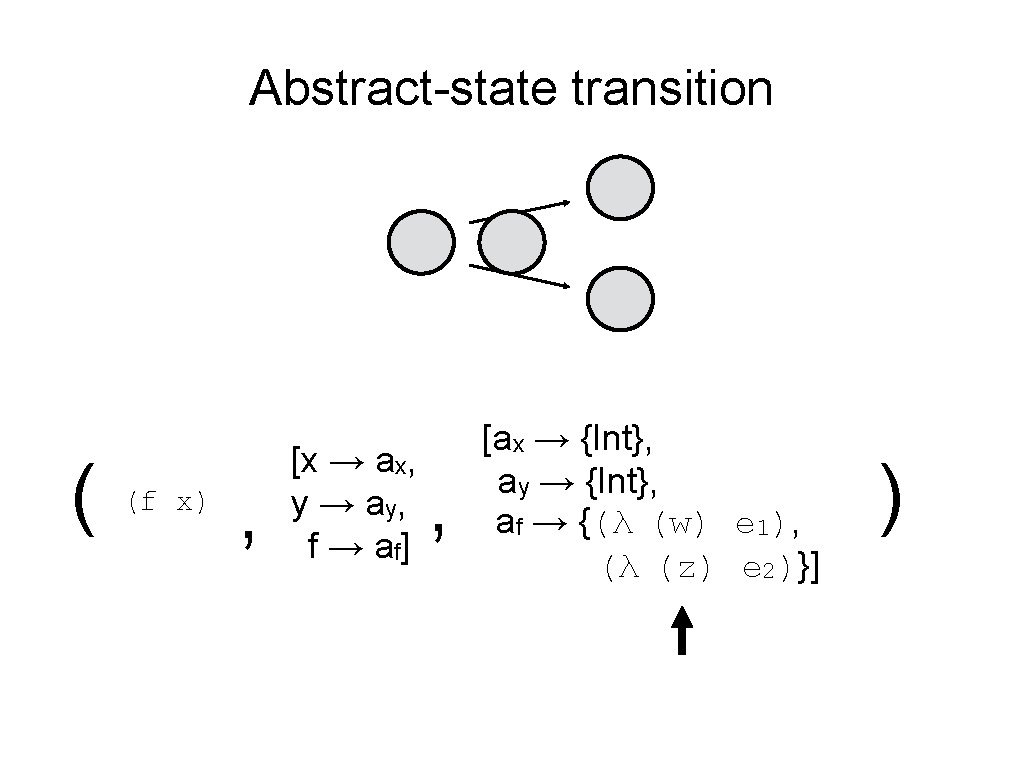Abstract-state transition ( (f x) , [x → ax, y → ay, f → a f] , [ax → {Int}, ay → {Int}, af → {(λ (w) e 1), (λ (z) e 2)}] )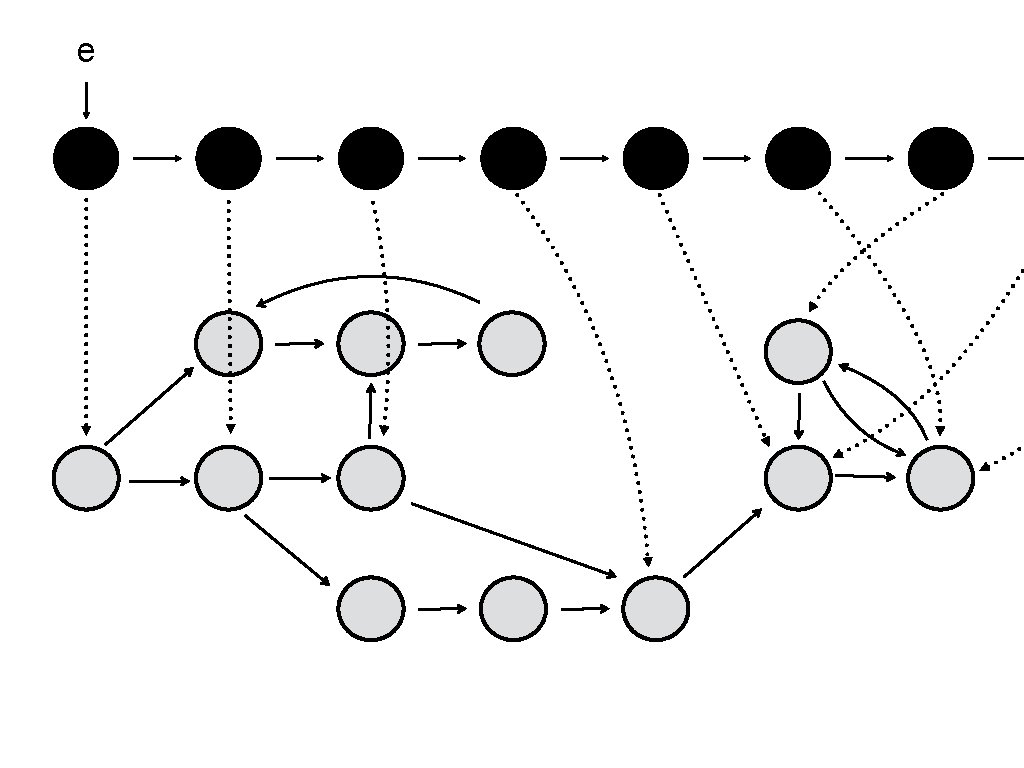e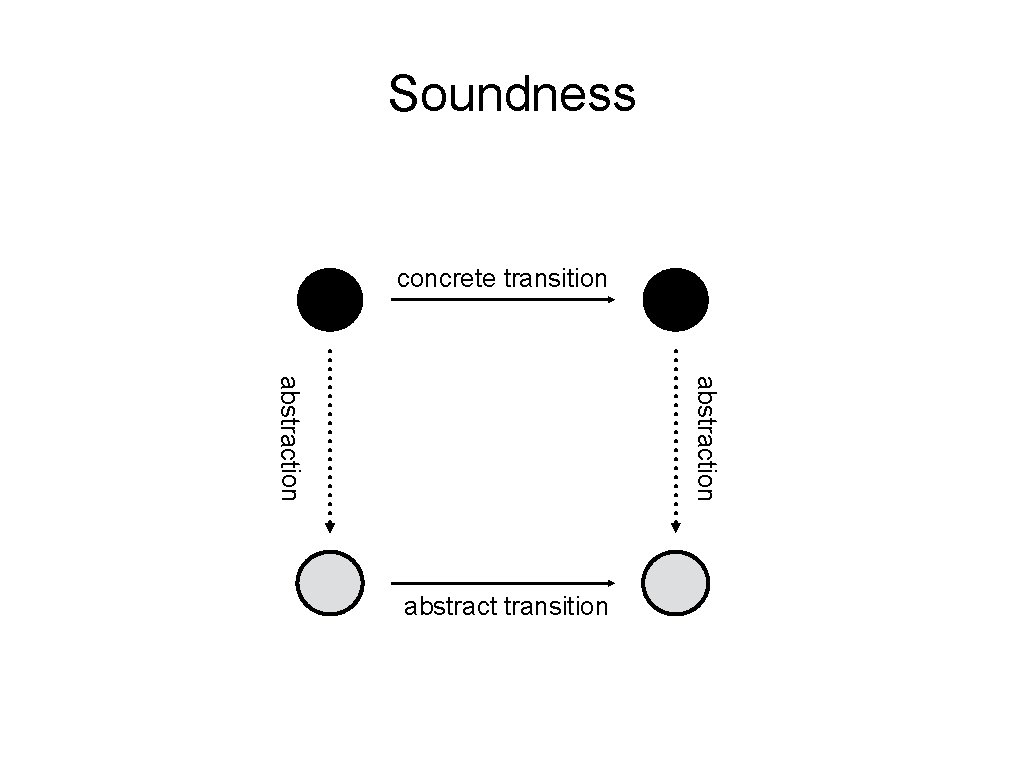Soundness concrete transition abstraction abstract transition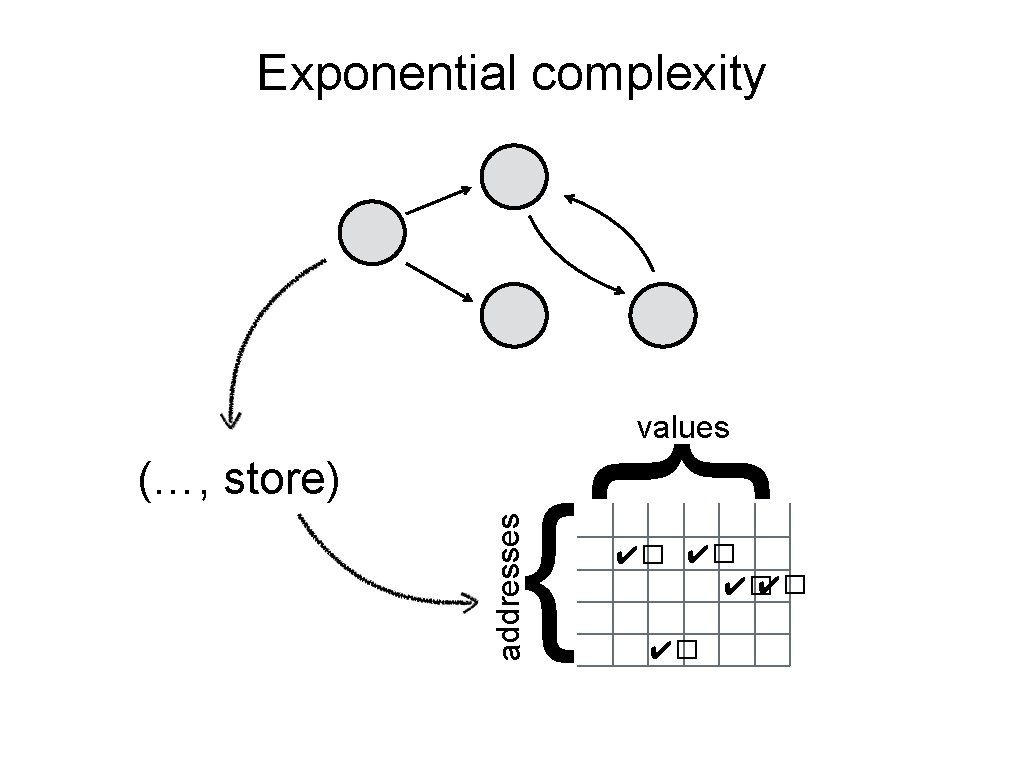Exponential complexity { addresses (…, store) { values ✔� ✔� ✔�Global store widening (flow-sensitive) ✔� ✔� … … … Control-flow graph … … ✔� ✔� ✔� per-point stores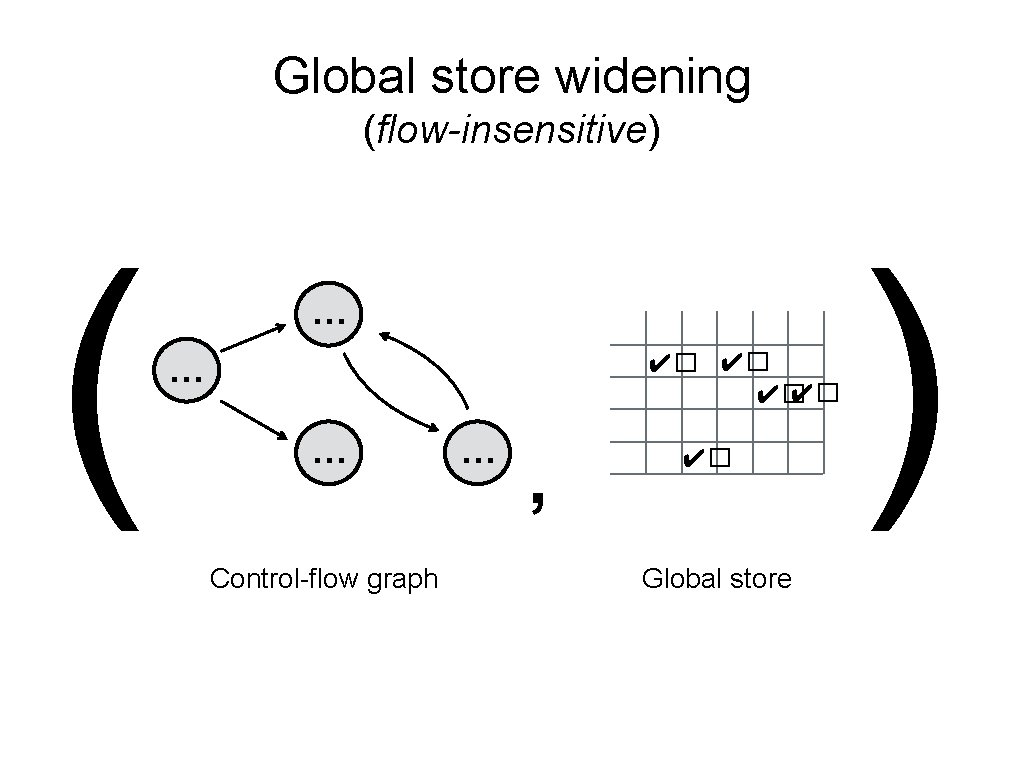Global store widening (flow-insensitive) ( … ✔� ✔� … … Control-flow graph … , ✔� Global store )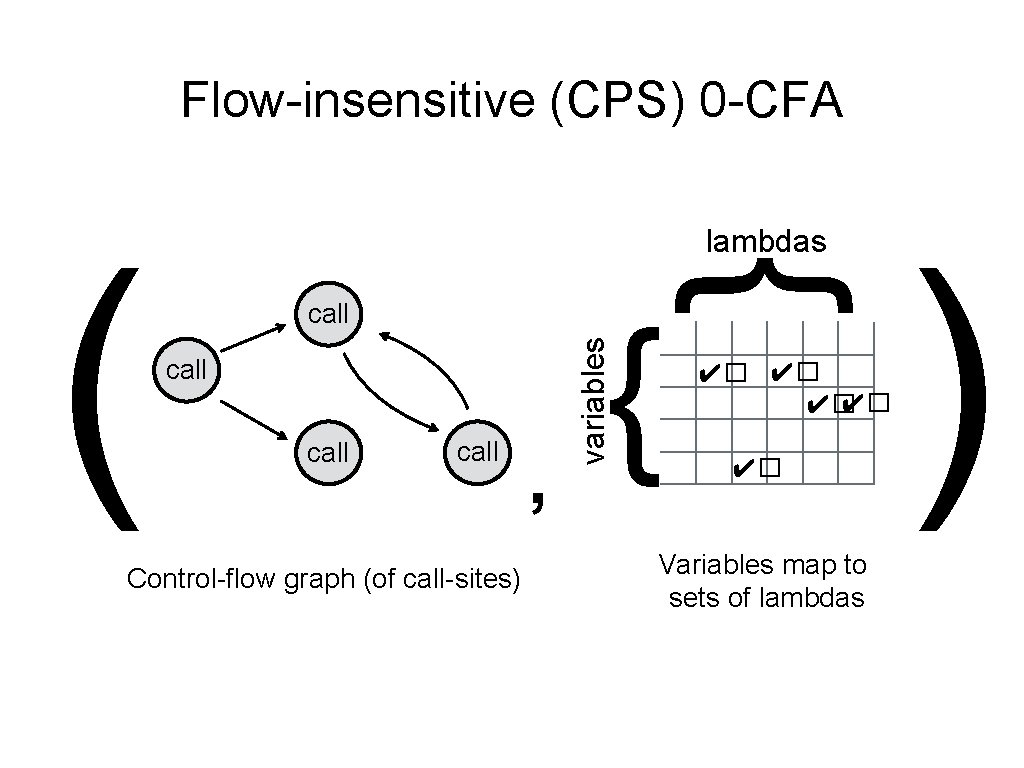Flow-insensitive (CPS) 0 -CFA call Control-flow graph (of call-sites) , { variables call { ( lambdas ✔� ✔� ✔� Variables map to sets of lambdas )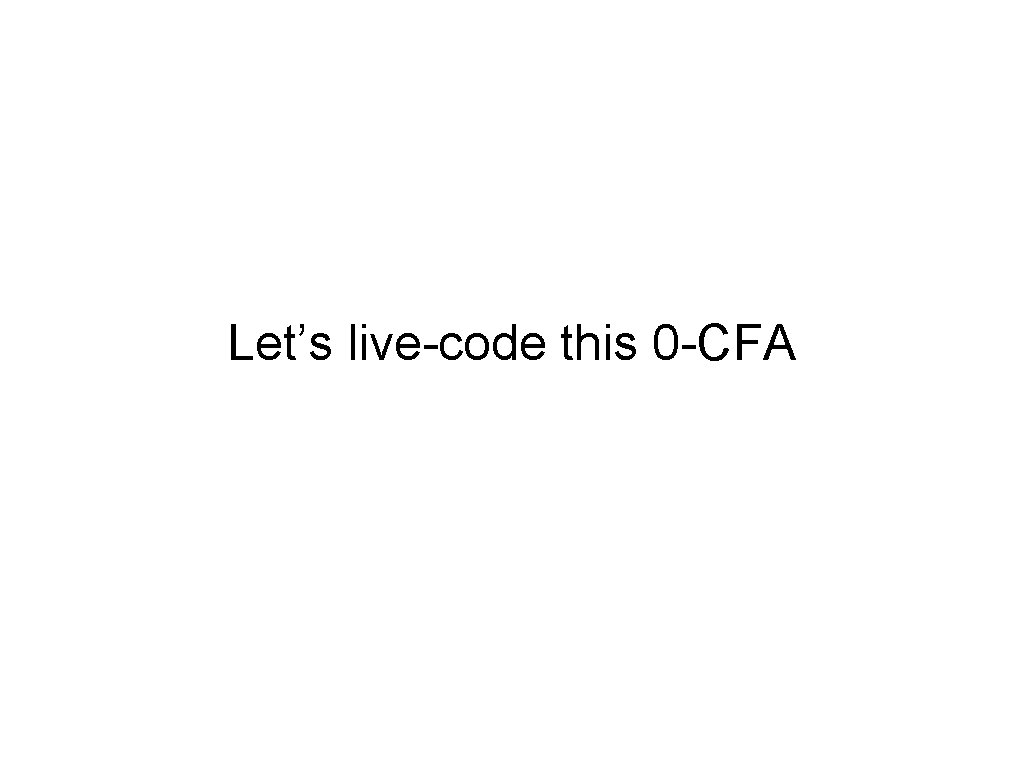Let’s live-code this 0 -CFA